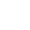# 醫療檢測的準確度：「偽陰性」、「偽陽性」到底是什麼意思？如何計算準確度？

2020/04/28 | | 標籤：
• 作者／林澤民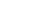### 「檢測準確度」與「統計檢定」概念可互相對應

 受檢者 不帶原 (non-carrier) 帶原 (true-carrier) 採檢結果 陽性 (positive) 偽陽性 (false positive) 真陽性 (true positive) 陰性 (negative) 真陰性 (true negative) 偽陰性 (false negative)

 虛無假設(H0) v. 研究假設(HA) 虛無假設為真 (H0 Ture) 研究假設為真 (HA True) 採檢結果 拒絕虛無假設 (positive) 型一錯誤 (size of test=α) 檢定強度 (power of test=1-β) 無法拒絕虛無假設 (negative) 信心水平 (1-α) 型二錯誤 (β)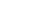### 數據科學中的「準確度」：F1 分數

F1 分數有時簡稱 F 分數，也稱為 Sørensen-Dice 係數。在數據科學裡，F1 常被用來做為搜尋、辨識「相似」資料準確度的度量。它可以用來衡量搜尋引擎的準確度，也常用在自然語言處理中資料的搜尋、辨識，當然它也可以用於人臉辨識。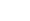F1 包含了兩個成分：召回率 (Recall) 和精密性 (Precision)。F1 是這兩個成分的平均數，但不是算數平均數而是調和平均數

$$F1=\frac{2}{\frac{1}{Recall}+\frac{1}{Precision}}$$

#### 那麼什麼是召回率？什麼是精密性？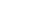$$Precision=\frac{(1-\beta )\pi }{\alpha+ (1-\alpha -\beta )\pi }$$

 ＼受檢者（人） 採檢結果＼ 不帶原 990 帶原 10 陽性 偽陽性 50* 真陽性 8 陰性 真陰性 940 偽陰性 2

*因 990 的 5%為 49.5 人不合常理，此處四捨五入

$$F1=\frac{2}{\frac{1}{Recall}+\frac{1}{Precision}}=\frac{2Recall}{1+Recall}$$

### 將 F1 應到到醫學檢驗上

$$F1=\frac{2}{\frac{1}{Recall}+\frac{1}{Precision}}=\frac{2(1-\beta )\pi }{\alpha+ (2-\alpha -\beta )\pi }$$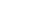### 後記：防疫中心數據核算

（2020/4/30 更新）

1. PCR（核酸檢測）：特異性=0.9999，敏感性=0.95，盛行率=0.0018 or 0.000016。對應於我所使用的統計學參數：α=0.0001，β=0.05，π=0.0018 or 0.000016。
2. 快篩：特異性=0.99，敏感性=0.75，盛行率=π=0.0018 or 0.000016。對應於我所使用的統計學參數：α=0.01，β=0.25，π=π=0.0018 or 0.000016。
• 講解中提到兩種盛行率：π=0.0018 以及 π=0.000016，前者被稱為「極大值」，後者為「合理值」。

• PCR 的精密性=0.9448
• 快篩 的精密性=0.1191• PCR的精密性=0.1319
• 快篩的精密性=0.0012

### 附錄：如何計算精密性——敏感性（真陽性）的反機率？

1. 把「行的條件機率」乘上「行的邊際機率」就可以得到「聯合機率」。
2. 把「聯合機率」除以「列的邊際機率」就可以得到「列的條件機率」。

 受檢者 不帶原 (non-carrier) 帶原 (true-carrier) 採檢結果 陽性 (positive) 偽陽性 α 真陽性 1 – β 陰性 (negative) 真陰性 1 – α 偽陰性 β 行的邊際機率 （隨機採檢人士帶原的先驗機率） 1 – π π

 受檢者 列的邊際機率 不帶原 (non-carrier) 帶原 (true-carrier) 採檢結果 陽性 (positive) 偽陽性 α(1 – π) 真陽性 (1 – β)π α + (1 – α – β)π 陰性 (negative) 真陰性 (1 – α)(1 – π) 偽陰性 βπ (1 – α) + (1 – α – β)π 行的邊際機率 （隨機採檢人士帶原的先驗機率） 1 – π π 1

 受檢者 列的邊際機率 不帶原 (non-carrier) 帶原 (true-carrier) 採檢結果 陽性 (positive) 偽陽性 $$\frac{\alpha (1-\pi) }{\alpha+ (1-\alpha -\beta )\pi }$$ 真陽性 $$\frac{(1-\beta) \pi }{\alpha+ (1-\alpha -\beta )\pi }$$ α + (1 – α – β)π 陰性 (negative) 真陰性 $$\frac{(1-\alpha) (1-\pi) }{(1-\alpha)+ (1-\alpha -\beta )\pi }$$ 偽陰性 $$\frac{\beta \pi }{(1-\alpha)+ (1-\alpha -\beta )\pi }$$ (1 – α) + (1 – α – β)π 行的邊際機率 （隨機採檢人士帶原的先驗機率） 1 – π π 1

$$Precision=\frac{(1-\beta )\pi }{\alpha+ (1-\alpha -\beta )\pi }$$

## 關於作者